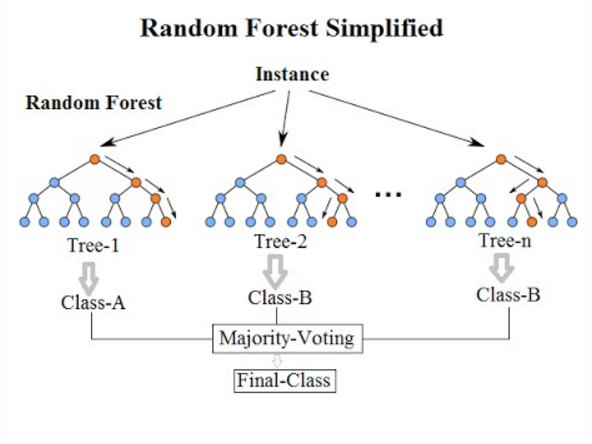Chapter 9 Titiannic Prediction with Random ForestCan’t see forest for the trees.

-- English Proverb

As we can see from the previous chapter, the decision tree models do not perform well in our prediction. In this chapter, we will try different models to see if we can improve the model’s accuracy by using Random Forest model.

Random Forest is one of the powerful ensembling machine learning algorithms which works by creating multiple decision trees and combining the output generated by each of the decision trees through a voting mechanism to produce an output based on the majority of decision trees’ votes (Trevor Hastie 2013). Figure 9.1 is an example of a random forest.Figure 9.1: Example of the Random Forest.

In the random forest, its decision tree classifier does not select all the data samples and attributes in each tree. Instead, every individual tree randomly selects data samples and attributes and combines the output at the end. The forest then removes the bias that a single decision tree might introduce in the model. The combination of the individual tree’s result is done in a vote carried out to find the result with the highest frequency. A test dataset is evaluated based on these outputs to get the final predicted results. Because of the evaluation process, the random forest model will have an estimated prediction accuracy once constructed. This models’ estimated accuracy can be used to compare between random forest models (Paluszyńska 2021).

References

Paluszyńska, Aleksandra. 2021. Understanding Random Forests with randomForestExplainer. https://cran.r-project.org/web/packages/randomForestExplainer/vignettes/randomForestExplainer.html.

Trevor Hastie, Jerome Friedman, Rob Tibshirani. 2013. Statistical Learning. 1st ed. https://www.statlearning.com/: Springer. https://doi.org/10.1007/978-1-4614-7138-7_2.